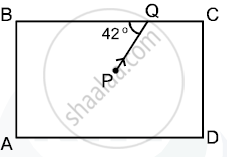# The Diagram Below Shows a Light Source P Embedded in a Rectangular Glass Block Abcd of Critical Angle 42°. Complete the Path of the Ray Pq Till It Emerges Out of the Block. - Physics

Short Note

The diagram below shows a light source P embedded in a rectangular glass block ABCD of critical angle 42°. Complete the path of the ray PQ till it emerges out of the block. [Write necessary angles].#### SolutionAt A
Angle of incidence = 38° >(ic
∴ TIR takes place
The ray is now incident on surface CD at B, hex angle of incidence = 42°
∴ Angle of refraction = 90°

Concept: Refraction of Light Through a Rectangular Glass Slab
Is there an error in this question or solution?